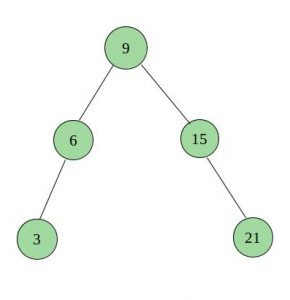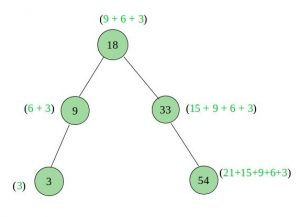Skip to content
Related Articles
BST to a Tree with sum of all smaller keys
• Difficulty Level : Easy
• Last Updated : 03 Nov, 2020

Given a Binary Search Tree(BST), convert it to a Binary Tree such that every key of the original BST is changed to key plus sum of all smaller keys in BST.

Given a BST with N Nodes we have to convert into Binary TreeGiven above BST with N=5 Nodes. The values at Node being 9, 6, 15, 3, 21

Binary Tree after convertionBinary Tree after convertion, the values at Node being 18, 9, 33, 3, 54

Solution: We will perform a regular Inorder traversal in which we keep track of sum of Nodes visited. Let this sum be sum. The Node which is being visited, add that key of Node to sum i.e. sum = sum + Node->key. Change the key of current Node to sum i.e. Node->key = sum.
When a BST is being traversed in inorder, for every key currently being visited, all keys that are already visited are all smaller keys.

## C++

 `// Program to change a BST to Binary Tree such ``// that key of a Node becomes original key plus ``// sum of all smaller keys in BST``#include `` ` `/* A BST Node has key, left child and ``   ``right child */``struct` `Node {``    ``int` `key;``    ``struct` `Node* left;``    ``struct` `Node* right;``};`` ` `/* Helper function that allocates a new ``   ``node with the given key and NULL left``   ``and right pointers.*/``struct` `Node* newNode(``int` `key)``{``    ``struct` `Node* node = ``new` `Node;``    ``node->key = key;``    ``node->left = NULL;``    ``node->right = NULL;``    ``return` `(node);``}`` ` `// A recursive function that traverses the``// given BST in inorder and for every key,``// adds all smaller keys to it``void` `addSmallerUtil(``struct` `Node* root, ``int``* sum)``{``    ``// Base Case``    ``if` `(root == NULL)``        ``return``;`` ` `    ``// Recur for left subtree first so that``    ``// sum of all smaller Nodes is stored``    ``addSmallerUtil(root->left, sum);`` ` `    ``// Update the value at sum``    ``*sum = *sum + root->key;`` ` `    ``// Update key of this Node``    ``root->key = *sum;`` ` `    ``// Recur for right subtree so that``    ``// the updated sum is added``    ``// to greater Nodes``    ``addSmallerUtil(root->right, sum);``}`` ` `// A wrapper over addSmallerUtil(). It ``// initializes sum and calls addSmallerUtil() ``// to recursively update and use value of``void` `addSmaller(``struct` `Node* root)``{``    ``int` `sum = 0;``    ``addSmallerUtil(root, &sum);``}`` ` `// A utility function to print inorder ``// traversal of Binary Tree``void` `printInorder(``struct` `Node* node)``{``    ``if` `(node == NULL)``        ``return``;``    ``printInorder(node->left);``    ``printf``(``"%d "``, node->key);``    ``printInorder(node->right);``}`` ` `// Driver program to test above function``int` `main()``{``    ``/* Create following BST``            ``9``            ``/ \``        ``6     15 */``    ``Node* root = newNode(9);``    ``root->left = newNode(6);``    ``root->right = newNode(15);`` ` `    ``printf``(``" Original BST\n"``);``    ``printInorder(root);`` ` `    ``addSmaller(root);`` ` `    ``printf``(``"\n BST To Binary Tree\n"``);``    ``printInorder(root);`` ` `    ``return` `0;``}`

## Java

 `// Java program to convert BST to binary tree ``// such that sum of all smaller keys is added ``// to every key`` ` `class` `Node {`` ` `    ``int` `data;``    ``Node left, right;`` ` `    ``Node(``int` `d)``    ``{``        ``data = d;``        ``left = right = ``null``;``    ``}``}`` ` `class` `Sum {`` ` `    ``int` `addvalue = ``0``;``}`` ` `class` `BSTtoBinaryTree {`` ` `    ``static` `Node root;``    ``Sum add = ``new` `Sum();`` ` `    ``// A recursive function that traverses ``    ``// the given BST in inorder and for every ``    ``// key, adds all smaller keys to it``    ``void` `addSmallerUtil(Node node, Sum sum)``    ``{`` ` `        ``// Base Case``        ``if` `(node == ``null``) {``            ``return``;``        ``}`` ` `        ``// Recur for left subtree first so that``        ``//  sum of all smaller Nodes is stored at sum``        ``addSmallerUtil(node.left, sum);`` ` `        ``// Update the value at sum``        ``sum.addvalue = sum.addvalue + node.data;`` ` `        ``// Update key of this Node``        ``node.data = sum.addvalue;`` ` `        ``// Recur for right subtree so that the ``        ``// updated sum is added to greater Nodes``        ``addSmallerUtil(node.right, sum);``    ``}`` ` `    ``// A wrapper over addSmallerUtil().  It ``    ``// initializes addvalue and calls``    ``// addSmallerUtil() to recursively update ``    ``// and use value of addvalue``    ``Node addSmaller(Node node)``    ``{``        ``addSmallerUtil(node, add);``        ``return` `node;``    ``}`` ` `    ``// A utility function to print inorder``    ``// traversal of Binary Tree``    ``void` `printInorder(Node node)``    ``{``        ``if` `(node == ``null``) {``            ``return``;``        ``}``        ``printInorder(node.left);``        ``System.out.print(node.data + ``" "``);``        ``printInorder(node.right);``    ``}`` ` `    ``// Driver program to test the above functions``    ``public` `static` `void` `main(String[] args)``    ``{``        ``BSTtoBinaryTree tree = ``new` `BSTtoBinaryTree();``        ``tree.root = ``new` `Node(``9``);``        ``tree.root.left = ``new` `Node(``6``);``        ``tree.root.right = ``new` `Node(``15``);`` ` `        ``System.out.println(``"Original BST"``);``        ``tree.printInorder(root);``        ``Node Node = tree.addSmaller(root);``        ``System.out.println(``""``);``        ``System.out.println(``"BST To Binary Tree"``);``        ``tree.printInorder(Node);``    ``}``}`

## Python3

 `# Program to change a BST to Binary Tree``# such that key of a Node becomes original ``# key plus sum of all smaller keys in BST`` ` `# A BST node has key, left child ``# and right child */``class` `Node: `` ` `    ``# Constructor to create a new node ``    ``def` `__init__(``self``, data): ``        ``self``.key ``=` `data ``        ``self``.left ``=` `None``        ``self``.right ``=` `None`` ` `# A recursive function that traverses the ``# given BST in inorder and for every key,``# adds all smaller keys to it ``def` `addSmallerUtil(root, ``Sum``):``     ` `    ``# Base Case ``    ``if` `root ``=``=` `None``: ``        ``return`` ` `    ``# Recur for left subtree first so that ``    ``# sum of all smaller Nodes is stored ``    ``addSmallerUtil(root.left, ``Sum``) `` ` `    ``# Update the value at sum ``    ``Sum``[``0``] ``=` `Sum``[``0``] ``+` `root.key `` ` `    ``# Update key of this Node ``    ``root.key ``=` `Sum``[``0``] `` ` `    ``# Recur for right subtree so ``    ``# that the updated sum is ``    ``# added to greater Nodes ``    ``addSmallerUtil(root.right, ``Sum``)`` ` `# A wrapper over addSmallerUtil(). It``# initializes sum and calls addSmallerUtil() ``# to recursively update and use value of``def` `addSmaller(root):``    ``Sum` `=` `[``0``] ``    ``addSmallerUtil(root, ``Sum``)``     ` `# A utility function to print``# inorder traversal of Binary Tree ``def` `printInorder(node):``    ``if` `node ``=``=` `None``: ``        ``return``    ``printInorder(node.left) ``    ``print``(node.key, end ``=` `" "``)``    ``printInorder(node.right)`` ` `# Driver Code``if` `__name__ ``=``=` `'__main__'``:``     ` `    ``# Create following BST ``    ``#         9 ``    ``#     / \ ``    ``#     6     15 ``    ``root ``=` `Node(``9``) ``    ``root.left ``=` `Node(``6``) ``    ``root.right ``=` `Node(``15``) `` ` `    ``print``(``"Original BST"``) ``    ``printInorder(root)``    ``print``()``    ``addSmaller(root) `` ` `    ``print``(``"BST To Binary Tree"``) ``    ``printInorder(root) `` ` `# This code is contributed by PranchalK`

## C#

 `using` `System;`` ` `// C#  program to convert BST to binary tree  ``// such that sum of all smaller keys is added  ``// to every key `` ` `public` `class` `Node``{`` ` `    ``public` `int` `data;``    ``public` `Node left, right;`` ` `    ``public` `Node(``int` `d)``    ``{``        ``data = d;``        ``left = right = ``null``;``    ``}``}`` ` `public` `class` `Sum``{`` ` `    ``public` `int` `addvalue = 0;``}`` ` `public` `class` `BSTtoBinaryTree``{`` ` `    ``public` `static` `Node root;``    ``public` `Sum add = ``new` `Sum();`` ` `    ``// A recursive function that traverses  ``    ``// the given BST in inorder and for every  ``    ``// key, adds all smaller keys to it ``    ``public` `virtual` `void` `addSmallerUtil(Node node, Sum sum)``    ``{`` ` `        ``// Base Case ``        ``if` `(node == ``null``)``        ``{``            ``return``;``        ``}`` ` `        ``// Recur for left subtree first so that ``        ``//  sum of all smaller Nodes is stored at sum ``        ``addSmallerUtil(node.left, sum);`` ` `        ``// Update the value at sum ``        ``sum.addvalue = sum.addvalue + node.data;`` ` `        ``// Update key of this Node ``        ``node.data = sum.addvalue;`` ` `        ``// Recur for right subtree so that the  ``        ``// updated sum is added to greater Nodes ``        ``addSmallerUtil(node.right, sum);``    ``}`` ` `    ``// A wrapper over addSmallerUtil().  It  ``    ``// initializes addvalue and calls ``    ``// addSmallerUtil() to recursively update  ``    ``// and use value of addvalue ``    ``public` `virtual` `Node addSmaller(Node node)``    ``{``        ``addSmallerUtil(node, add);``        ``return` `node;``    ``}`` ` `    ``// A utility function to print inorder ``    ``// traversal of Binary Tree ``    ``public` `virtual` `void` `printInorder(Node node)``    ``{``        ``if` `(node == ``null``)``        ``{``            ``return``;``        ``}``        ``printInorder(node.left);``        ``Console.Write(node.data + ``" "``);``        ``printInorder(node.right);``    ``}`` ` `    ``// Driver program to test the above functions ``    ``public` `static` `void` `Main(``string``[] args)``    ``{``        ``BSTtoBinaryTree tree = ``new` `BSTtoBinaryTree();``        ``BSTtoBinaryTree.root = ``new` `Node(9);``        ``BSTtoBinaryTree.root.left = ``new` `Node(6);``        ``BSTtoBinaryTree.root.right = ``new` `Node(15);`` ` `        ``Console.WriteLine(``"Original BST"``);``        ``tree.printInorder(root);``        ``Node Node = tree.addSmaller(root);``        ``Console.WriteLine(``""``);``        ``Console.WriteLine(``"BST To Binary Tree"``);``        ``tree.printInorder(Node);``    ``}``}`` ` `  ``// This code is contributed by Shrikant13`

Output:
```Original BST
6 9 15
BST To Binary Tree
6 15 30
```

Attention reader! Don’t stop learning now. Get hold of all the important DSA concepts with the DSA Self Paced Course at a student-friendly price and become industry ready.  To complete your preparation from learning a language to DS Algo and many more,  please refer Complete Interview Preparation Course.

In case you wish to attend live classes with industry experts, please refer DSA Live Classes

My Personal Notes arrow_drop_up